Q:

# Print the Longest Bitonic Subsequence

Given an array you have to print the longest bitonic subsequence from the array. A bitonic sequence is a sequence where numbers of that sequence are sorted and they are increasing first then decreasing.

Note: A sorted increasing sequence is also a bitonic sequence and similarly sorted decreasing sequence is a bitonic sequence.

```    Test Case T
T no. of lines with the value of N and corresponding values.
E.g.
3
5
1 4 2 5 7

8
1 7 3 2 5 4 8 1

17
1 10 9 3 5 4 11 7 4 8 9 6 19 5 7 8 2

Constrains:
1≤ T ≤ 10
1≤ N ≤ 100
1≤ A[i] ≤ 1000

Output:
Print the bitonic subsequence.
```

Example

```    T=3
N=5
1 4 2 5 7
1 2 5 7

N=8
1 7 3 2 5 4 8 1
1 7 3 2 1

N=17
1 10 9 3 5 4 11 7 4 8 9 6 19 5 7 8 2
1 3 4 7 8 9 6 5 2```

For this sequence: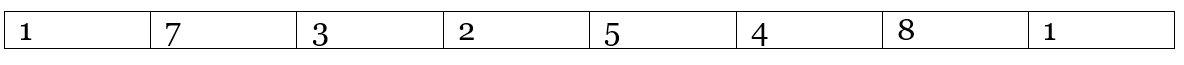Longest increasing subsequence from front: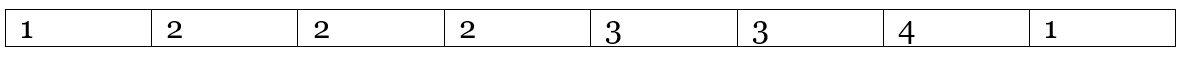Longest increasing subsequence from rear: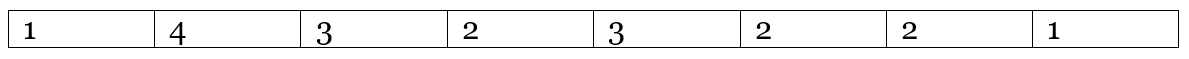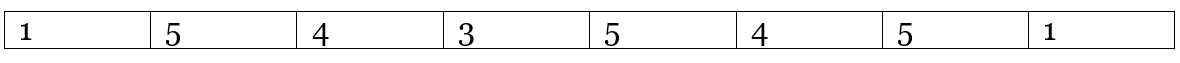Therefore, the length of the longest bitonic subsequence is 5.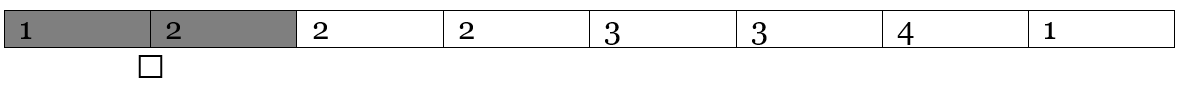Therefore, the numbers from the first LIS are: 1 7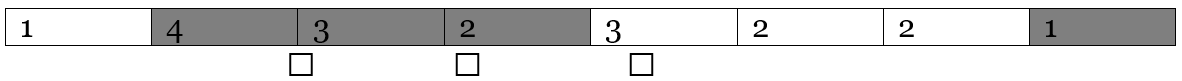Therefore, the numbers from the second LIS are: 3 2 1
Therefore, the sequence will be 1 7 3 2 1

C++ implementation:

``````#include <bits/stdc++.h>
using namespace std;

void print_biotonic_sequences(int* f, int* l, int ind, int* a, int n)
{
int i = ind;
int j = ind;

list<int> lst;
lst.push_back(a[ind]);

while (i >= 0) {
//taking the ith element which have a diffencence of one from ind
if (a[i] < a[ind] && (f[i] + 1 == f[ind])) {
lst.push_front(a[i]);
ind = i;
}
i--;
}

ind = j;

while (j < n) {
if (a[ind] > a[j] && (l[j] + 1 == l[ind])) {
lst.push_back(a[j]);
ind = j;
}
j++;
}

list<int>::iterator it;

//print the values
cout << "The values are : ";
for (it = lst.begin(); it != lst.end(); it++) {
cout << *it << " ";
}
cout << endl;
}

int main()
{
int t;

cout << "Test Case : ";
cin >> t;

while (t--) {
int n;

cout << "Number of elements : ";
cin >> n;

int a[n];

cout << "Enter the elements : ";
for (int i = 0; i < n; i++) {
cin >> a[i];
}

int f[n], l[n];
//initiate the new arrays
for (int i = 0; i < n; i++) {
f[i] = 1;
l[i] = 1;
}

//calculate the LIS from front
for (int i = 1; i < n; i++) {
for (int j = 0; j < i; j++) {
if (a[j] < a[i])
f[i] = max(f[i], f[j] + 1);
}
}

//calculate the LIS from behind
for (int i = n - 2; i >= 0; i--) {
for (int j = n - 1; j > i; j--) {
if (a[j] < a[i])
l[i] = max(l[i], l[j] + 1);
}
}

int m = 0;
int store = 0;

//adding the two arrays to join them
for (int i = 0; i < n; i++) {
if (m < (f[i] + l[i] - 1)) {
store = i;
m = f[i] + l[i] - 1;
}
}
print_biotonic_sequences(f, l, store, a, n);
}
return 0;
}
``````

Output

```Test Case : 3
Number of elements : 5
Enter the elements : 1 4 2 5 7
The sequence is : 1 2 5 7
Number of elements : 8
Enter the elements : 1 7 3 2 5 4 8 1
The sequence is : 1 7 3 2 1
Number of elements : 17
Enter the elements : 1 10 9 3 5 4 11 7 4 8 9 6 19 5 7 8 2
The sequence is : 1 3 4 7 8 9 6 5 2```